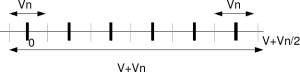- DSP log - http://www.dsplog.com -

Understanding Shannon’s capacity equation

Posted By Krishna Sankar On June 15, 2008 @ 7:25 pm In Channel | 13 Comments

Let us try to understand the formula for Channel Capacity with an Average Power Limitation, described in Section 25 of the landmark paper A Mathematical Theory for Communication , by Mr. Claude Shannon .

Further, the following writeup is based on Section 12.5.1 from Fundamentals of Communication Systems by John G. Proakis, Masoud SalehiSimple example with voltage levels

Let us consider that we have two voltage sources:
(a) Signal source which can generate voltages in the range to volts
(b) Noise source which can generate voltage levels to volts.Figure: Discrete voltage levels with noise

Let us now try to send information at discrete voltage levels from the source (thick black lines as shown in the above figure). It is intuitive to guess that the receiver will be able to decode the received symbol correctly if the received signal lies within .

So, the number of different discrete voltages levels (information) which can be sent, while ensuring error free communication is the total voltage level divided by the noise voltage level i.e.
.

Extending to Gaussian channel

Let us transmit randomly chosen discrete voltage levels meeting the average power constraint,

, where is the signal power.

The noise signal follows the Gaussian probability distribution function

with mean and variance .

The noise power is,

.

The average total (signal plus noise) voltage over symbols is .

Similiarly, the average noise voltage over symbols is .

Combining the above two equations, the number of different messages which can be ‘reliably transmitted‘ is,

.

Note:

1. The product of the signal and noise accumulated over many symbols average to zero, i.e

.

2. Since the noise is Gaussian distributed, the noise can theoretically go from to . So the above result cannot ensure zero probability of error in receiver, but only arbitrarily small probability of error.

Converting to bits per transmission

With different messages, the number of bits which can be transmitted per transmission is,

bits/transmission.

Bringing bandwidth into the equation

Let us assume that the available bandwidth is .

Noise is of power spectral density spread over the bandwidth . So the noise power in terms of power spectral density and bandwidth is,

.

From our previous post on transmit pulse shaping filter  that minimum required bandwidth for transmitting symbols with symbol period isHz. Conversely, if the available bandwidth is , the maximum symbol rate (transmissions per second) is .

Multiplying the equation for bits per transmission with transmission per second of and replacing the noise term , the capacity is

bits/second.

Voila! This is Shannon’s equation for capacity of band limited additive white Gaussian noise channel with an average transmit power constraint.References

[COMM-SYS-PROAKIS-SALEHI] Fundamentals of Communication Systems by John G. Proakis, Masoud SalehiURL to article: http://www.dsplog.com/2008/06/15/shannon-gaussian-channel-capacity-equation/

URLs in this post:

 A Mathematical Theory for Communication: http://plan9.bell-labs.com/cm/ms/what/shannonday/shannon1948.pdf

 Mr. Claude Shannon: http://en.wikipedia.org/wiki/Claude_Shannon

 Fundamentals of Communication Systems by John G. Proakis, Masoud Salehi: http://www.amazon.com/gp/redirect.html?ie=UTF8&location=http%3A%2F%2Fwww.amazon.com%2FFundamentals-Communication-Systems-John-Proakis%2Fdp%2F013147135X&tag=dl04-20&linkCode=ur2&camp=1789&creative=9325

 transmit pulse shaping filter: http://www.dsplog.com/2008/04/14/transmit-pulse-shape-nyquist-sinc-rectangular/